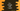# C++ program to print a triangle with 1 and 0## C++ program to print a triangle with 1 and 0:

In this post, I will show you how to create one triangle with 1 and 0 in C++. The final triangle will look as like below :

``````1
1 0
1 0 1
1 0 1 0
1 0 1 0 1``````

This is a common interview question and asked mostly to find out if the candidate can design a program with multiple loops.

We need two loops to print these types of patterns. One loop will work inside another loop. The outer loop is used to point to the rows or lines of the triangle and the inner loop is used to print the numbers.

We will take the size of the triangle as input from the user. Based on this input, we will run one for loop from 0 to size - 1. *For each iteration of the loop, we will run one more loop to print the *1 0 values.

Let’s check the program:

### C++ program:

Below is the complete C++ program:

``````#include <iostream>

using namespace std;

int main()
{
int height;
cout << "Enter the height of the triangle :" << endl;
cin >> height;
cout << endl;

for (int i = 0; i < height; i++)
{
for (int j = 0; j <= i; j++)
{
j % 2 == 0 ? cout << "1 " : cout << "0 ";
}
cout << endl;
}

return 0;
}``````

### Explanation :

• height integer variable is used to store the height of the triangle. We are taking this input from the user.
• The outer for loop is running from i = 0 to i = height - 1
• The inner for loop is running from j = 0 to j = i
• Inside the inner loop, we are checking if the value of j is divisible by 2 or not. If yes it prints 1, else it prints 0. i.e. for index 0, 2, 4… it prints 1 and for index 1, 3, 5… it prints 0.

### Sample Output :

``````Enter the height of the triangle :
5

1
1 0
1 0 1
1 0 1 0
1 0 1 0 1

Enter the height of the triangle :
10

1
1 0
1 0 1
1 0 1 0
1 0 1 0 1
1 0 1 0 1 0
1 0 1 0 1 0 1
1 0 1 0 1 0 1 0
1 0 1 0 1 0 1 0 1
1 0 1 0 1 0 1 0 1 0``````